Year 8 Interactive Maths - Second Edition

## Scale Drawings

A map cannot be of the same size as the area it represents.  So, the measurements are scaled down to make the map of a size that can be conveniently used by users such as motorists, cyclists and bushwalkers.  A scale drawing of a building (or bridge) has the same shape as the real building (or bridge) that it represents but a different size.  Builders use scaled drawings to make buildings and bridges.

A ratio is used in scale drawings of maps and buildings.  That is: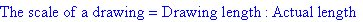Likewise, we have:A scale is usually expressed in one of two ways:

• using units as in 1 cm to 1 km
• without explicitly mentioning units as in 1 : 100 000.
###### Note:

A scale of 1 : 100 000 means that the real distance is 100 000 times the length of 1 unit on the map or drawing.

#### Example 14

Write the scale 1 cm to 1 m in ratio form.

##### Solution:#### Example 15

Simplify the scale 5 mm : 1 m.

##### Solution:#### Example 16

Simplify the scale 5 cm : 2 km.

##### Solution: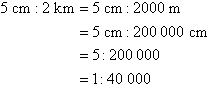### Calculating the Actual Distance using the Scale

If the scale is 1 : x, then multiply the map distance by x to calculate the actual distance.

#### Example 17

A particular map shows a scale of 1 : 5000.  What is the actual distance if the map distance is 8 cm?

##### Solution: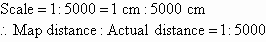Map distance = 8 cm

Let the actual distance be a cm.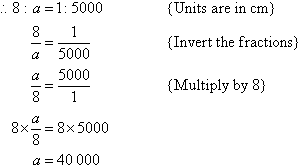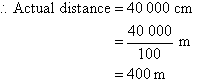###### Alternative Way:

Map distance = 8 cm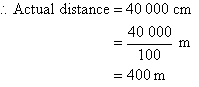### Calculating the Scaled Distance using the Actual Distance

If the scale is 1 : x, then divide the actual distance by x to calculate the map distance.

#### Example 18

A particular map shows a scale of 1 cm : 5 km.  What would the map distance (in cm) be if the actual distance is 14 km?

##### Solution: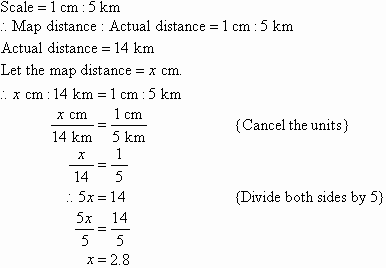So, the map distance is 2.8 cm.

###### Alternative Way: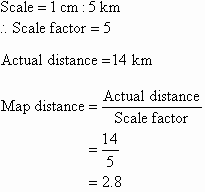So, the map distance is 2.8 cm.